Question

# For each of the variables described below, indicate whether it is a quantitative or a categorical...

For each of the variables described below, indicate whether it is a quantitative or a categorical (qualitative) variable. Also, indicate the level of measurement for the variable: nominal, ordinal, interval, or ratio. Make sure your responses are the most specific possible.

Variable Type of variable Level of measurement (a) Dosage (in milligrams) of medication Quantitative Nominal Ordinal Categorical Interval Ratio

(b) Customer satisfaction rating (very satisfied, somewhat satisfied, somewhat dissatisfied, or very dissatisfied) Quantitative Nominal Ordinal Categorical Interval Ratio

(c) Gender Quantitative Nominal Ordinal Categorical Interval Ratio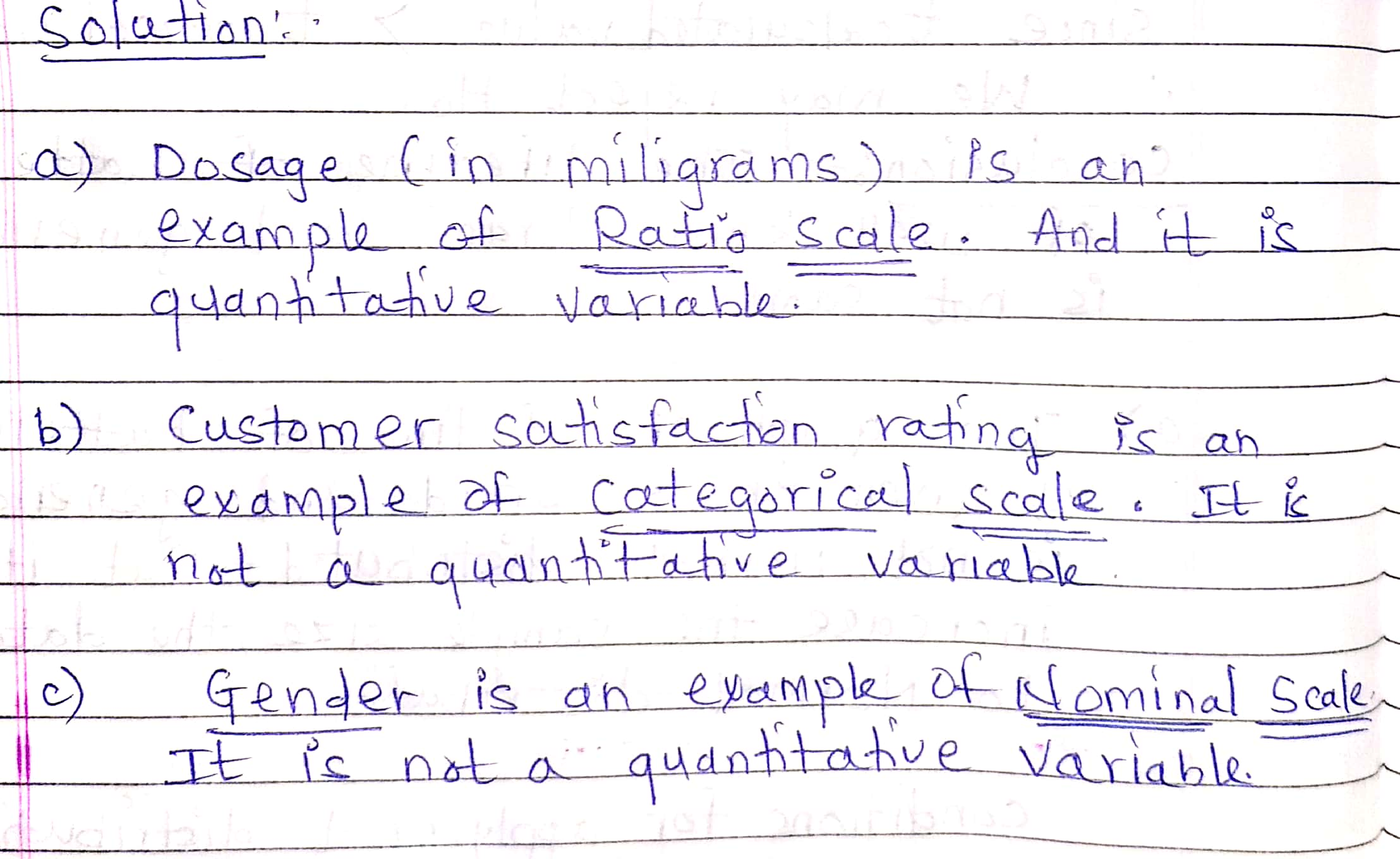#### Earn Coins

Coins can be redeemed for fabulous gifts.

Similar Homework Help Questions
• ### 10 11 For each of the variables described below, indicate whether it is a quantitative or...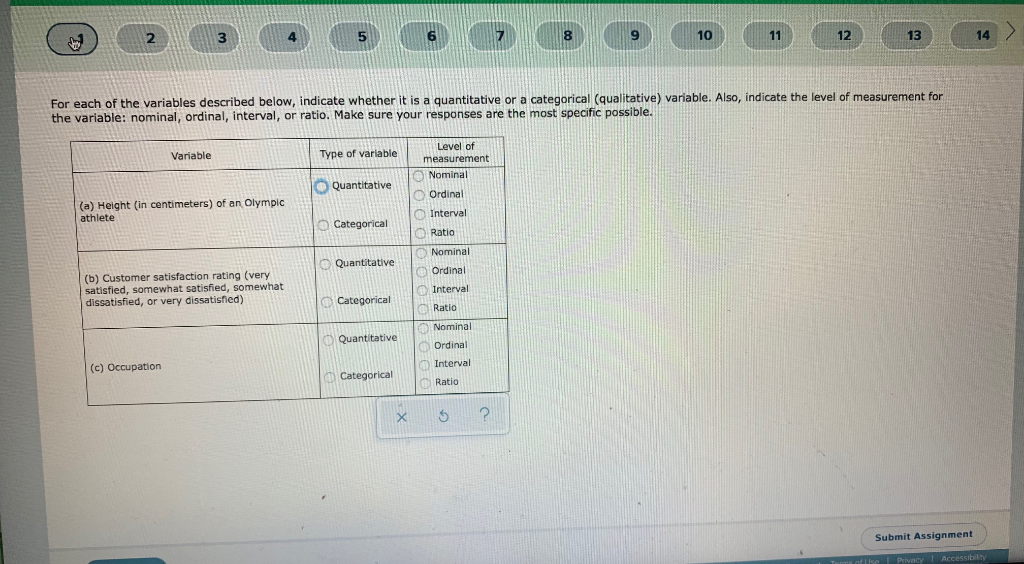10 11 For each of the variables described below, indicate whether it is a quantitative or a categorical (qualitative) variable. Also, indicate the level of measurement for the variable: nominal, ordinal, interval, or ratio. Make sure your responses are the most specific possible. Variable Type of variable Level of measurement Nominal Ordinal Quantitative (a) Height (in centimeters) of an Olympic athlete Categorical Interval Ratio Quantitative Nominal Ordinal (b) Customer satisfaction rating (very satisfied, somewhat satisfied, somewhat dissatisfied, or very dissatisfied)...

• ### For each of the variables described below, indicate whether it is a quantitative or a categorical...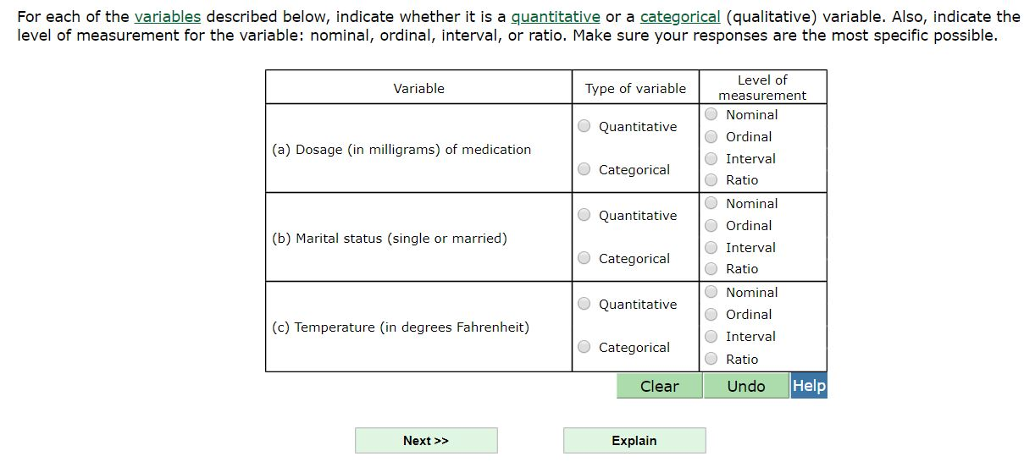For each of the variables described below, indicate whether it is a quantitative or a categorical (qualitative) variable. Also, indicate the level of measurement for the variable: nominal, ordinal, interval, or ratio. Make sure your responses are the most specific possible Level of measurement Nominal Ordinal Interval Ratio Variable Type of variable Quantitative Categorical O Quantitative Categorical O Quantitative (a) Dosage (in milligrams) of medication O Nominal O Ordinal O Interval O Ratio (b) Marital status (single or married) Nominal...

• ### For each of the variables described below, indicate whether it is a quantitative or a categorical...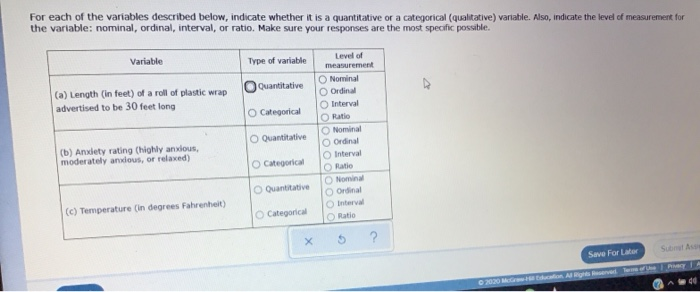For each of the variables described below, indicate whether it is a quantitative or a categorical (qualitative) variable. Also, indicate the level of measurement for the variable: nominal, ordinal, interval, or ratio. Make sure your responses are the most specific possible. Variable Type of variable Quantitative (a) Length (in feet) of a roll of plastic wrap advertised to be 30 feet long O Categorical Quantitative Level of measurement Nominal Ordinal Interval O Ratio Nominal Ordinal Interval Ratio Nominal Ordinal Inter...

• ### For each of the variables described below, indicate whether it is a quantitative or a categorical...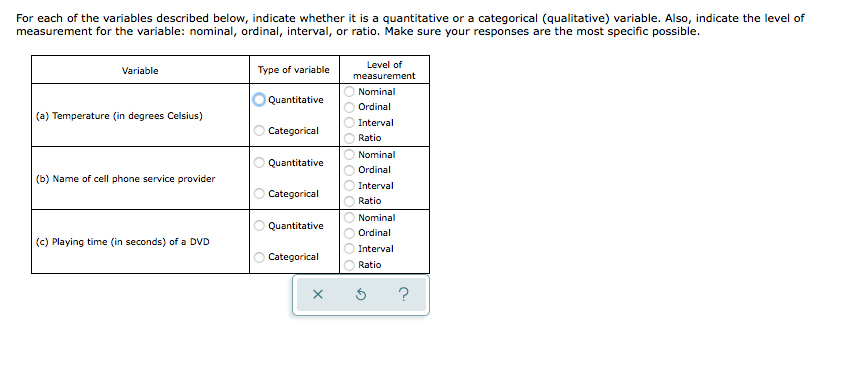For each of the variables described below, indicate whether it is a quantitative or a categorical (qualitative) variable. Also, indicate the level of measurement for the variable: nominal, ordinal, interval, or ratio. Make sure your responses are the most specific possible. Variable Type of variable Quantitative (a) Temperature (in degrees Celsius) Categorical Quantitative Level of measurement Nominal Ordinal Interval Ratio Nominal Ordinal Interval Ratio Nominal Ordinal Interval Ratio (b) Name of cell phone service provider Categorical OOOOOOOOO Quantitative (c) Playing...

• ### For each of the variables described below, indicate whether it is a quantitative or a categorical...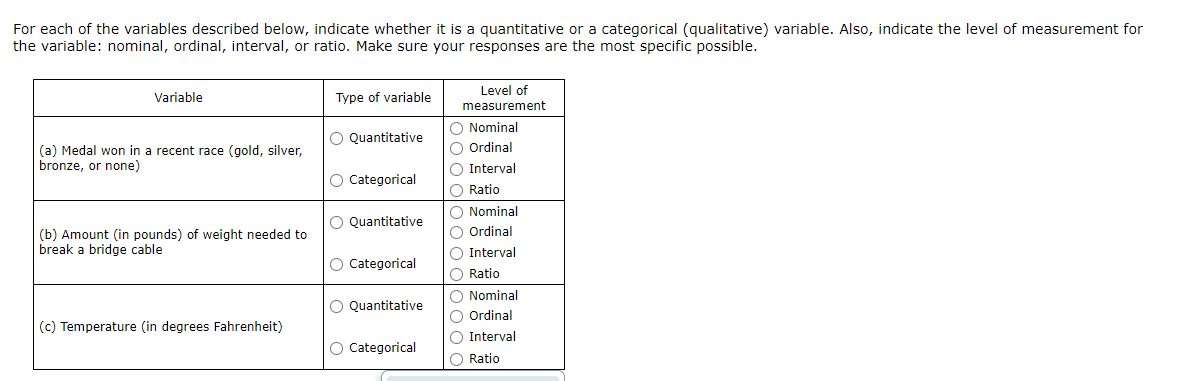For each of the variables described below, indicate whether it is a quantitative or a categorical (qualitative) variable. Also, indicate the level of measurement for the variable: nominal, ordinal, interval, or ratio. Make sure your responses are the most specific possible. Variable Type of variable O Quantitative (a) Medal won in a recent race (gold, silver, bronze, or none) O Categorical O Quantitative Level of measurement O Nominal Ordinal Interval O Ratio O Nominal Ordinal Interval Ratio Nominal O Ordinal...

• ### For each of the variables described below, indicate whether it is a quantitative or a categorical...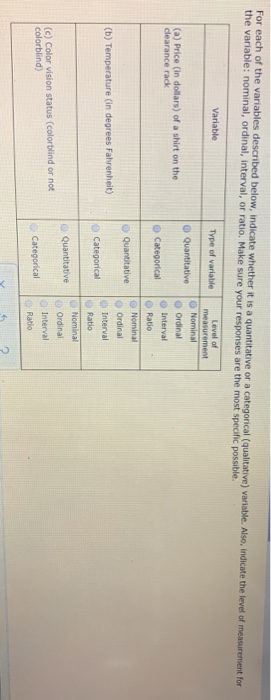For each of the variables described below, indicate whether it is a quantitative or a categorical (qualitative) variable. Also, indicate the level of measurement for the variable: nominal, ordinal, interval, or ratio. Make sure your responses are the most specific possible Variable Type of variable Level of measurement Nominal O Quantitative Ordinal (a) Price (in dollars) of a shirt on the clearance rack Interval Categorical Ratio Quantitative (b) Temperature (in degrees Fahrenheit) Categorical Nominal Ordinal Interval Ratio Nominal Ordinal Interval...

• ### Classify the following data. Indicate whether the data is qualitative or quantitative, indicate whether the data...

Classify the following data. Indicate whether the data is qualitative or quantitative, indicate whether the data is discrete, continuous, or neither, and indicate the level of measurement for the data. A survey response to "Do you favor a flat tax?" 1) Yes 2) No 1. Are these data qualitative or quantitative? 2. Are these data discrete, continuous or neither? 3. What is the highest level of measurement the data possesses? Nominal, ordinal, interval, or ratio?

• ### Classify the variables A to F as qualitative or quantitative. For quantitative data, please state whether...

Classify the variables A to F as qualitative or quantitative. For quantitative data, please state whether the variable is discrete or continuous. Then, for each one, distinguish between the four (4) levels of measurement (nominal, ordinal, interval and ratio). What is the total cost of textbooks for your courses? How many times do you see your Academic Advisor during the semester? How do you rate the Add/Drop period? Very good/ Good/ Bad

• ### Classify the variables A to F as qualitative or quantitative. For quantitative data, please state whether...

Classify the variables A to F as qualitative or quantitative. For quantitative data, please state whether the variable is discrete or continuous. Then, for each one, distinguish between the four (4) levels of measurement (nominal, ordinal, interval and ratio). What is your Year of Graduation (YOG)? How many courses are you enrolled in? What method of payment do you use to pay for textbooks? Cash Credit Card Debit Card

• ### A mortgage bank randomly samples the accounts of its timeshare customers. Indicate if each of the...

A mortgage bank randomly samples the accounts of its timeshare customers. Indicate if each of the following variables is categorical or numerical. If it is categorical, indicate the level of measurement. If it is numerical, is it discrete or continuous? a. The original purchase price of a customer's timeshare unit. b. The place of residence of a timeshare owner. c. The degree of satisfaction of a timeshare owner with the maintenance of the unit purchased (from 1: very dissatisfied to...